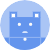search
Search
menu search toc more_vert
Guest 0reps
Thanks for the thanks!
close
account_circle
Profile
exit_to_app
Sign out
help Ask a question
search
keyboard_voice
close
Searching Tips
Search for a recipe:
"Creating a table in MySQL"
Search for an API documentation: "@append"
Search for code: "!dataframe"
Apply a tag filter: "#python"
Useful Shortcuts
/ to open search panel
Esc to close search panel
to navigate between search results
d to clear all current filters
Enter to expand content previewDoc SearchCode Search BetaSORRY NOTHING FOUND!
mic
Start speaking...Voice search is only supported in Safari and Chrome.
Shrink
Navigate to
A
A
brightness_medium
share
arrow_backShareTwitterFacebook

# NumPy | array method

NumPy
chevron_right
Documentation
schedule Jul 1, 2022
Last updated
local_offer PythonNumPy
Tags

Numpy's `array(~)` method constructs a Numpy array out of the provided object, which is typically a list or a tuple.

# Parameters

1. `object` | `array-like objects`

Data source used to build a Numpy array. Typically, we use a list or a tuple.

2. `dtype`link | `string` or `type` | `optional`

Type of data stored in the Numpy array. By default, type will be inferred.

3. `ndmin`link | `int` | `optional`

The minimum number of dimensions the Numpy array will have.

# Return value

A Numpy array with the data-type as specified.

# Examples

## Creating Numpy array using list

``` np.array([1,2,3]) array([1, 2, 3]) ```

The inferred type of the data is `int`.

## Creating Numpy array using tuple

``` np.array((1,2,3)) array([1, 2, 3]) ```

## Creating Numpy array with explicit type

``` np.array((1,2,3), float)np.array((1,2,3), "float") array([1., 2., 3.]) ```

Notice how we have `1.` instead of just `1` - this means that the data in the Numpy array is of type `float` instead of `int`. You can also provide the `dtype` in string form, like `"float"`.

## Creating 2D Numpy array

``` np.array([[1, 2, 3], [4, 5, 6]]) array([[1, 2, 3],       [4, 5, 6]]) ```

## Creating 2D Numpy array using ndmin

``` np.array([1, 2], ndmin=2) array([[1, 2]]) ```

Here, normally we would have a 1D Numpy array, but since we specified `ndmin=2`, we get a 2D Numpy array instead.

mail